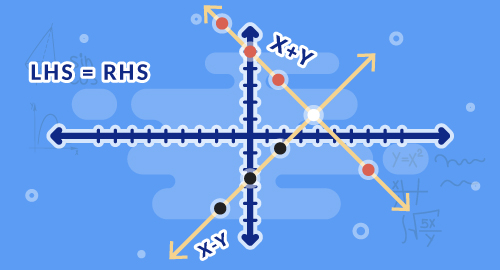#### Need Help?

Get in touch with usSAT/ACT

# Analyze and Solve Linear Equations

Maths

Solve different linear equation that has only one side variable, combining like terms, both side variable. Solve linear equations in one variable with one solution, infinitely many solution, and no solution. Compare two different proportional relationship represented in different ways. Derive the equation y=mx for a line passing through origin and the equation y=mx+b for a line intercepting the vertical axis at b.

##### Course Duration
•3 Weeks9 Sessions
•50 mins per session
##### Tutor## What you'll learn

• 1

Solve equations that have like terms on one side.

• 2

Solve equations that have variables on both the sides of equal to sign.

• 3

Determine the number of solutions for an equation.

• 4

Compare proportional relationship represented in different ways.

• 5

Understand the slope of the line.

• 6

Write equations to describe linear relationship.

• 7

Find y-intercept of the graph.

• 8

Derive the equation y=mx+b.

## Skills you'll learnProblem solvingAnalytical thinking